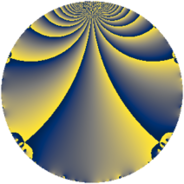# Properties

 Label 399.2.aLevel $399$ Weight $2$ Character orbit 399.a Rep. character $\chi_{399}(1,\cdot)$ Character field $\Q$ Dimension $19$ Newform subspaces $7$ Sturm bound $106$ Trace bound $3$

# Related objects

## Defining parameters

 Level: $$N$$ $$=$$ $$399 = 3 \cdot 7 \cdot 19$$ Weight: $$k$$ $$=$$ $$2$$ Character orbit: $$[\chi]$$ $$=$$ 399.a (trivial) Character field: $$\Q$$ Newform subspaces: $$7$$ Sturm bound: $$106$$ Trace bound: $$3$$ Distinguishing $$T_p$$: $$2$$, $$5$$

## Dimensions

The following table gives the dimensions of various subspaces of $$M_{2}(\Gamma_0(399))$$.

Total New Old
Modular forms 56 19 37
Cusp forms 49 19 30
Eisenstein series 7 0 7

The following table gives the dimensions of the cuspidal new subspaces with specified eigenvalues for the Atkin-Lehner operators and the Fricke involution.

$$3$$$$7$$$$19$$FrickeDim.
$$+$$$$+$$$$+$$$$+$$$$1$$
$$+$$$$+$$$$-$$$$-$$$$3$$
$$+$$$$-$$$$+$$$$-$$$$5$$
$$+$$$$-$$$$-$$$$+$$$$1$$
$$-$$$$+$$$$+$$$$-$$$$4$$
$$-$$$$-$$$$-$$$$-$$$$5$$
Plus space$$+$$$$2$$
Minus space$$-$$$$17$$

## Trace form

 $$19q + 5q^{2} - q^{3} + 21q^{4} + 10q^{5} - 3q^{6} + 3q^{7} + 9q^{8} + 19q^{9} + O(q^{10})$$ $$19q + 5q^{2} - q^{3} + 21q^{4} + 10q^{5} - 3q^{6} + 3q^{7} + 9q^{8} + 19q^{9} + 6q^{10} + 4q^{11} + 9q^{12} + 10q^{13} + q^{14} - 6q^{15} + 29q^{16} + 22q^{17} + 5q^{18} - q^{19} - 2q^{20} - q^{21} - 12q^{22} - 8q^{23} - 15q^{24} + 29q^{25} - 18q^{26} - q^{27} + 5q^{28} + 18q^{29} + 6q^{30} - 7q^{32} - 4q^{33} - 14q^{34} - 6q^{35} + 21q^{36} + 2q^{37} - 3q^{38} + 18q^{39} - 18q^{40} + 22q^{41} + q^{42} + 36q^{43} - 4q^{44} + 10q^{45} - 16q^{46} - 8q^{47} + q^{48} + 19q^{49} - 37q^{50} - 2q^{51} - 10q^{52} - 6q^{53} - 3q^{54} - 24q^{55} + 21q^{56} + 3q^{57} - 50q^{58} + 4q^{59} - 42q^{60} + 10q^{61} - 56q^{62} + 3q^{63} + 29q^{64} + 28q^{65} + 4q^{66} - 20q^{67} + 2q^{68} - 16q^{69} - 18q^{70} - 40q^{71} + 9q^{72} + 30q^{73} - 18q^{74} + 17q^{75} - 7q^{76} + 4q^{77} - 34q^{78} + 16q^{79} - 98q^{80} + 19q^{81} - 70q^{82} - 52q^{83} - 7q^{84} - 44q^{85} - 68q^{86} - 14q^{87} - 108q^{88} + 38q^{89} + 6q^{90} - 6q^{91} - 88q^{92} - 48q^{94} - 6q^{95} - 63q^{96} - 26q^{97} + 5q^{98} + 4q^{99} + O(q^{100})$$

## Decomposition of $$S_{2}^{\mathrm{new}}(\Gamma_0(399))$$ into newform subspaces

Label Dim. $$A$$ Field CM Traces A-L signs $q$-expansion
$$a_2$$ $$a_3$$ $$a_5$$ $$a_7$$ 3 7 19
399.2.a.a $$1$$ $$3.186$$ $$\Q$$ None $$-1$$ $$-1$$ $$0$$ $$1$$ $$+$$ $$-$$ $$-$$ $$q-q^{2}-q^{3}-q^{4}+q^{6}+q^{7}+3q^{8}+\cdots$$
399.2.a.b $$1$$ $$3.186$$ $$\Q$$ None $$-1$$ $$1$$ $$4$$ $$-1$$ $$-$$ $$+$$ $$+$$ $$q-q^{2}+q^{3}-q^{4}+4q^{5}-q^{6}-q^{7}+\cdots$$
399.2.a.c $$1$$ $$3.186$$ $$\Q$$ None $$1$$ $$-1$$ $$0$$ $$-1$$ $$+$$ $$+$$ $$+$$ $$q+q^{2}-q^{3}-q^{4}-q^{6}-q^{7}-3q^{8}+\cdots$$
399.2.a.d $$3$$ $$3.186$$ 3.3.148.1 None $$1$$ $$-3$$ $$4$$ $$-3$$ $$+$$ $$+$$ $$-$$ $$q+\beta _{1}q^{2}-q^{3}+(\beta _{1}+\beta _{2})q^{4}+(1+\beta _{1}+\cdots)q^{5}+\cdots$$
399.2.a.e $$3$$ $$3.186$$ 3.3.404.1 None $$1$$ $$3$$ $$0$$ $$-3$$ $$-$$ $$+$$ $$+$$ $$q+\beta _{2}q^{2}+q^{3}+(3+\beta _{1}-\beta _{2})q^{4}+(-\beta _{1}+\cdots)q^{5}+\cdots$$
399.2.a.f $$5$$ $$3.186$$ 5.5.1240016.1 None $$1$$ $$5$$ $$-2$$ $$5$$ $$-$$ $$-$$ $$-$$ $$q+\beta _{3}q^{2}+q^{3}+(1+\beta _{4})q^{4}+\beta _{2}q^{5}+\cdots$$
399.2.a.g $$5$$ $$3.186$$ 5.5.368464.1 None $$3$$ $$-5$$ $$4$$ $$5$$ $$+$$ $$-$$ $$+$$ $$q+(1-\beta _{1})q^{2}-q^{3}+(2-\beta _{1}+\beta _{2})q^{4}+\cdots$$

## Decomposition of $$S_{2}^{\mathrm{old}}(\Gamma_0(399))$$ into lower level spaces

$$S_{2}^{\mathrm{old}}(\Gamma_0(399)) \cong$$ $$S_{2}^{\mathrm{new}}(\Gamma_0(19))$$$$^{\oplus 4}$$$$\oplus$$$$S_{2}^{\mathrm{new}}(\Gamma_0(21))$$$$^{\oplus 2}$$$$\oplus$$$$S_{2}^{\mathrm{new}}(\Gamma_0(57))$$$$^{\oplus 2}$$$$\oplus$$$$S_{2}^{\mathrm{new}}(\Gamma_0(133))$$$$^{\oplus 2}$$